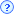< P <相对湿度=
%含湿量=
g(水蒸汽)/kg(干空气)露点温度=湿球温度=干球温度=比容=比焓=内能=逸度系数=

×